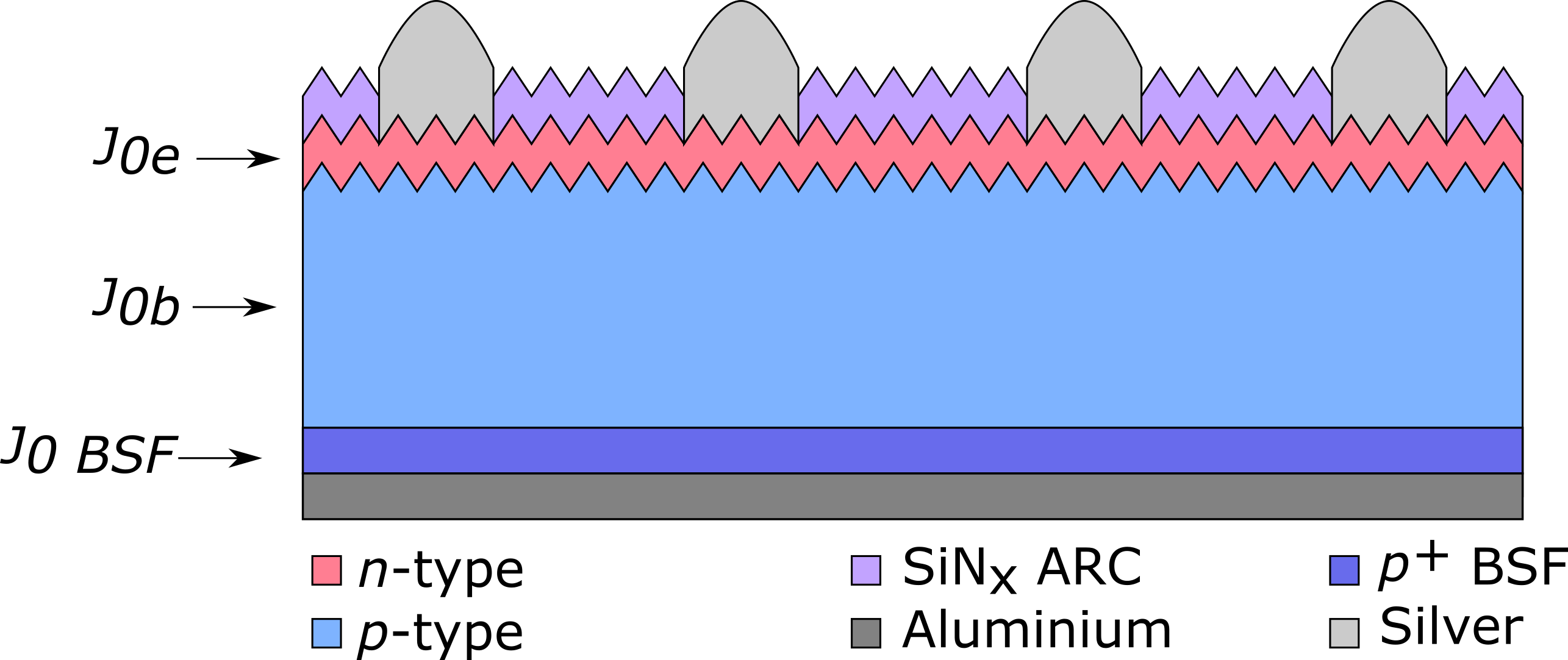# Recombination currents in a solar cell

Recombination occurs spatially throughout the solar cell in varying magnitudes. The total recombination within the device can be thought of as the sum of each individual recombination component, which are distinguished by the location at which the recombination occurs. The thermal recombination current J0 is one such conceptualisation of the electron and hole recombination which can be used to quantify regional recombination components .   For a typical aluminium back surface field (Al-BSF) solar cell, the common recombination components are:

1. J0e – recombination which occurs in the emitter.
2. J0b – Recombination which occurs in the base or undiffused bulk of the solar cell.
3. J0bsf – recombination which occurs in the diffused back surface field of the device.

These recombination currents are depicted schematically in Figure 1 below.Figure 1: Schematic illustration of the recombination processes in a standard aluminum back surface field silicon solar cell.

When researching and designing optimised solar cells, it is often imperative to identify the individual loss mechanisms within the device to, for instance, assess the effect of process variations or simulate device performance. The recombination in the diffused region can dominate a device’s performance and therefore the measurement of the emitter recombination by the extraction of the emitter saturation current density J0e is commonly performed using photoconductance measurements. In this procedure, a symmetrically passivated and diffused sample is fabricated with ideally identical process conditions to the actual devices of interest. For  symmetrically passivated and diffused sample, provided that the sample is in high injection, the effective lifetime is written as :$\frac{1}{\tau_{eff}}=\left(\ \frac{1}{\tau_{intrinsic}}+\frac{1}{\tau_{SRH}}\right)+J_{0e}\frac{2\left(N_b+\mathrm{\Delta n}\right)}{qWn_i^2}$, (1)

where Nb is the dopant concentration in the bulk, W is the width of the sample, ni is the intrinsic carrier concentration, Δn is the excess carrier density and Nb is the doping level in the bulk. Assuming that τSRH is independent of Δn, differentiating with respect to Δn yields the form,$J_{0e}=\frac{1}{2}qn_i^2W\frac{d}{d\mathrm{\Delta n}}\left(\frac{1}{\tau_{eff}}-\frac{1}{\tau_{intrinsic}}-\frac{1}{\tau_{SRH}}\right)$,  (2)

which enables the extraction of J0 from the linear portion of the slope of 1/τeff1/τintrinsic vs. Δn. The bracketed term in the RHS of Eq. 2.57 is often referred to as the corrected lifetime, τcorr, and accounts for Auger recombination in the bulk. The 1/τSRH component accounts for SRH processes in the bulk, and in the case of high lifetime material (such as Float-zone silicon) is often assumed to be negligible. This method is commonly referred to as the “Kane-Swanson method”, and is a simple, quick and powerful method for the extraction of J0. For symmetrically diffused and/or passivated samples, J0 is analogous to J0e or J0s respectively, provided that in the latter case the sample is under strong inversion or accumulation. In the original 1985 paper, Kane and Swanson outlined key assumptions affecting the accuracy of this method;

• Assumed uniform excess carrier density in the bulk, which allows the average generation rate in the bulk to be approximated as a uniform generation rate in the sample. This is not strictly correct due to finite carrier mobility within the sample. Kane and Swanson recommend reducing the sample width and minimising the surface recombination velocity to minimise this effect.
• ni is constant, and therefore not taking into account Band gap narrowing effects with increasing injection.
• The sample must be in high injection, as in this region the bulk region has a linear dependence on injection level, whereas the surface has a quadratic dependence. As a rule of thumb, Δnmin > 10Nb was suggested.
• The sample must not be limited by Auger or SRH recombination in the bulk in high injection; the bulk lifetime is constant.
• The diffused region is electrically “invisible” and contributes negligibly to the conductance measurement.

A good analogy is to look at a solar cell as a leaking bucket where the water level represents the open circuit voltage and the holes in the bucket are the various recombination processes. This is shown schematically in the animation below.